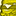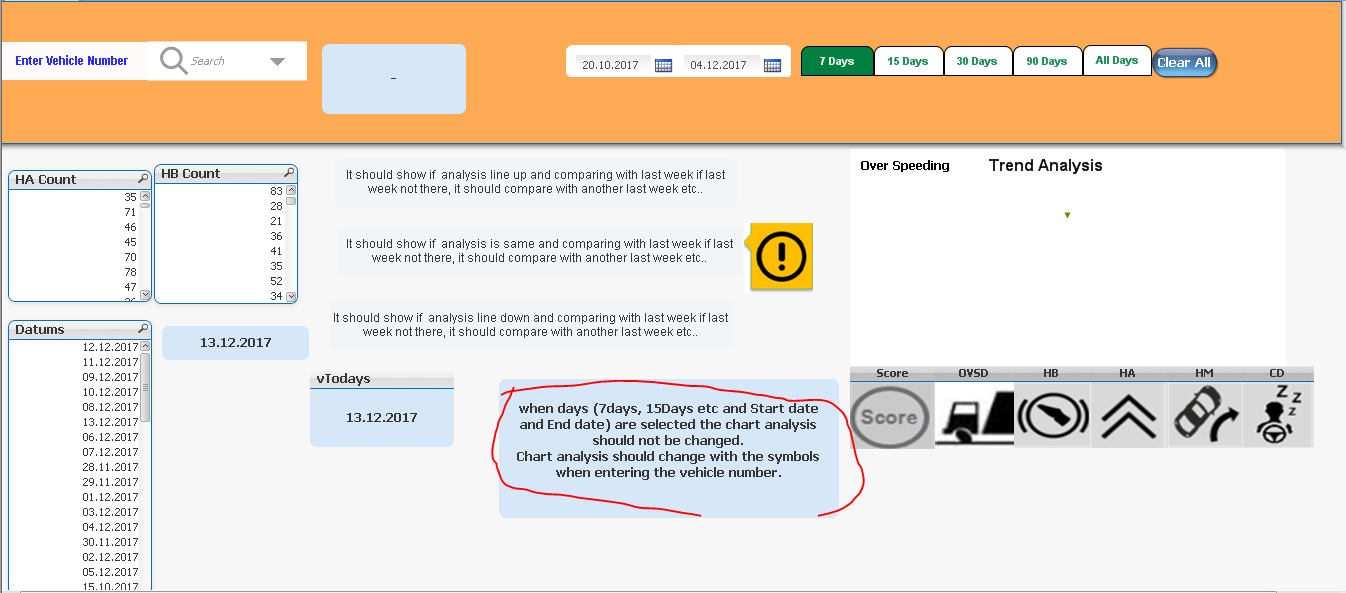# QlikView App Dev

Discussion Board for collaboration related to QlikView App Development.

Announcements
cancel
Showing results for
Did you mean:Creator III

## show and hide set analysis

Hi

Please find the attached QVW and how can achieve those things.

Can please anyone solve this one.

7 RepliesMVP

I tried to find the issue, but I am not sure I understand... I looked at thisWhat is 'It' here? "It should not reflect...."? Do I need to look at something else also?Creator III
Author

Hi,

Thanks for your quick response sunny.Contributor III

Hi Kumar,

I'm not sure that understood right.

But It seems that alternate states options should work for your case.Creator III
Author

Hi Venelin and sunny,

I resolved that issue with putting that field name in the chart expression like field Date=,

But the problem is with symbols should be changed along with analysis for Score, OVSD, HB, HA,HM,CD.

For this can i take each one 3 symbols?

and how to implement the IF condition in that Layout of image text object If last week is not there.

(weeks are 45,46,49).According my condition 49th week is comparing with 48th week but there is no 48 week data. How to resolve this?Creator III
Author

Hi ,

Thanks for your reply. I wrote this one  in object layout. Please let me know this if condition is correct or not.

=IF(vscore=0,1,0)

=IF(ceil( sum({\$<WEEK={\$(=Max(WEEK)-1)}>}\$(sumkm))/sum({\$<WEEK={\$(=Max(WEEK)-1)}>}[Kms Travelled])*1000)<>0,

if(ceil( sum({\$<WEEK={\$(=Max(WEEK))}>} \$(sumkm))/sum({\$<WEEK={\$(=Max(WEEK))}>}[Kms Travelled])*1000)

<

ceil( sum({\$<WEEK={\$(=Max(WEEK)-1)}>}\$(sumkm))/sum({\$<WEEK={\$(=Max(WEEK)-1)}>}[Kms Travelled])*1000), 1,

IF(ceil( sum({\$<WEEK={\$(=Max(WEEK)-1)}>}\$(sumkm))/sum({\$<WEEK={\$(=Max(WEEK)-1)}>}[Kms Travelled])*1000)=0 and

ceil( sum({\$<WEEK={\$(=Max(WEEK)-2)}>}\$(sumkm))/sum({\$<WEEK={\$(=Max(WEEK)-2)}>}[Kms Travelled])*1000)<>0,

IF(ceil( sum({\$<WEEK={\$(=Max(WEEK))}>} \$(sumkm))/sum({\$<WEEK={\$(=Max(WEEK))}>}[Kms Travelled])*1000)

< ceil( sum({\$<WEEK={\$(=Max(WEEK)-2)}>}\$(sumkm))/sum({\$<WEEK={\$(=Max(WEEK)-2)}>}[Kms Travelled])*1000),1,

IF(ceil( sum({\$<WEEK={\$(=Max(WEEK)-2)}>}\$(sumkm))/sum({\$<WEEK={\$(=Max(WEEK)-2)}>}[Kms Travelled])*1000)=0 and

ceil( sum({\$<WEEK={\$(=Max(WEEK)-3)}>}\$(sumkm))/sum({\$<WEEK={\$(=Max(WEEK)-3)}>}[Kms Travelled])*1000)<>0,

IF(ceil( sum({\$<WEEK={\$(=Max(WEEK))}>} \$(sumkm))/sum({\$<WEEK={\$(=Max(WEEK))}>}[Kms Travelled])*1000)

< ceil( sum({\$<WEEK={\$(=Max(WEEK)-3)}>}\$(sumkm))/sum({\$<WEEK={\$(=Max(WEEK)-3)}>}[Kms Travelled])*1000),1,0))))))

Is this expression correct?Specialist II

Why do you give something like 2 if condition one after another ? If you use the below only the first if condition will be checked is that what you want? by right you should use only the 2 if condition for week comparison

=If(vhb= 0,1,0)
=
if(ceil( sum({\$<WEEK={\$(=Max(WEEK))}>}[HB Count])/sum({\$<WEEK={\$(=Max(WEEK))}>}[Kms Travelled])*1000)
=
ceil( sum({\$<WEEK={\$(=Max(WEEK)-1)}>}[HB Count])/sum({\$<WEEK={\$(=Max(WEEK)-1)}>}[Kms Travelled])*1000), 1,0)Creator III
Author

The Same IF condition i put for comparing the current week and last week. But its taking continues  weeks (43,42,41,40...).

If suppose current week is 43 and last week 40 (there is no data in 42,41) when iam putting that condition its comparing with 42week.

But its should not happen. It should compare with 40 which is having data.

These three conditions for three symbols to show. IF its '=' below one

=if(ceil( sum({\$<WEEK={\$(=Max(WEEK))}>}[HB Count])/sum({\$<WEEK={\$(=Max(WEEK))}>}[Kms Travelled])*1000)
=
ceil( sum({\$<WEEK={\$(=Max(WEEK)-1)}>}[HB Count])/sum({\$<WEEK={\$(=Max(WEEK)-1)}>}[Kms Travelled])*1000), 1,0)

If Current week is greater than  last week

=if(ceil( sum({\$<WEEK={\$(=Max(WEEK))}>}[HB Count])/sum({\$<WEEK={\$(=Max(WEEK))}>}[Kms Travelled])*1000)
>
ceil( sum({\$<WEEK={\$(=Max(WEEK)-1)}>}[HB Count])/sum({\$<WEEK={\$(=Max(WEEK)-1)}>}[Kms Travelled])*1000), 1,0)

If current week less than last week.

=if(ceil( sum({\$<WEEK={\$(=Max(WEEK))}>}[HB Count])/sum({\$<WEEK={\$(=Max(WEEK))}>}[Kms Travelled])*1000)
<
ceil( sum({\$<WEEK={\$(=Max(WEEK)-1)}>}[HB Count])/sum({\$<WEEK={\$(=Max(WEEK)-1)}>}[Kms Travelled])*1000), 1,0)

What will do in this scenario?